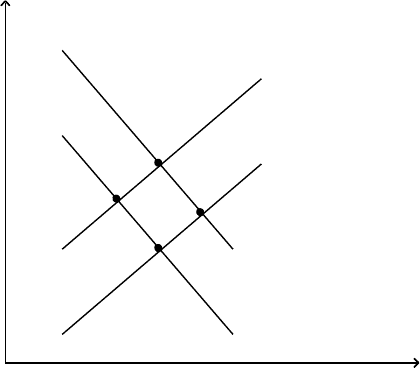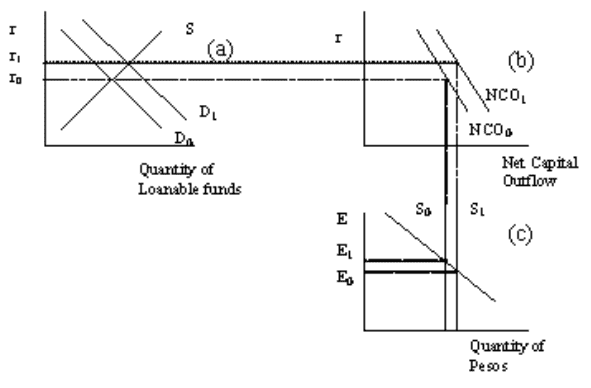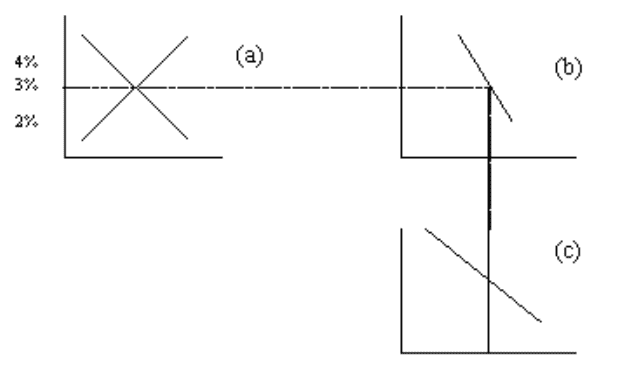Study Guides (400,000)
US (230,000)
UMD (10,000)
ECON (900)
Sarna (4)
Final

# ECON 201 Study Guide - Final Guide: Exchange Rate, Loanable Funds, Foreign Exchange Market

Department
Economics
Course Code
ECON 201
Professor
Sarna
Study Guide
Final

This preview shows pages 1-3. to view the full 11 pages of the document.UMD Macro Exam 2 (ID P)
Multiple Choice
Identify the choice that best completes the statement or answers the question.
____ 1. When a country experiences capital flight its
a. net capital outflow increases and its real exchange rate rises.
b. net capital outflow increases and its real exchange rate falls.
c. net capital outflow decreases and its real exchange rate rises.
d. net capital outflow decreases and its real exchange rate falls.
____ 2. If Kenya experienced capital flight, the supply of Kenyan schillings in the market for foreign-currency
exchange would shift
a. left, which would make the real exchange rate of the Kenyan schilling appreciate.
b. left, which would make the real exchange rate of the Kenyan schilling depreciate.
c. right, which would make the real exchange rate of the Kenyan schilling appreciate.
d. right, which would make the real exchange rate of the Kenyan schilling depreciate.
Figure 26-3. The figure shows two demand-for-loanable-funds curves and two supply-of-loanable-funds
curves.
A
B
C
F
S
S
D
D
1
2
1
2
i
____ 3. Refer to Figure 26-3. Which of the following movements would be consistent with the government budget
going from deficit to surplus and the simultaneous enactment of an investment tax credit?
a. a movement from Point A to Point C
b. a movement from Point B to Point A
c. a movement from Point B to Point F
d. a movement from Point C to Point B
____ 4. Refer to Figure 26-3. What, specifically, does the label on the vertical axis, i, represent?
a. the nominal interest rate
b. the real interest rate

Only pages 1-3 are available for preview. Some parts have been intentionally blurred.c. the inflation rate
d. the dividend yield
____ 5. Refer to Figure 26-3. Which of the following movements shows the effects of the government going from a
budget deficit to a budget surplus?
a. a movement from Point A to Point B
b. a movement from Point B to Point A
c. a movement from Point A to Point F
d. a movement from Point C to Point B
____ 6. The price of a basket of goods and services in the U.S. is \$600. In Canada the same basket of goods costs 700
Canadian dollars. If the nominal exchange rate were 1.2 Canadian dollars per U.S. dollar, what would be the
real exchange rate?
a. 700/600
b. 600/700
c. 700/720
d. None of the above is correct.
The diagram below represents the market for loanable funds and the market for foreign-currency exchange in
Mexico. Use the diagram to answer the following questions.
Figure 32-7
____ 7. Refer to Figure 32-7. Which of the following is consistent with capital flight from Mexico?
a. The real exchange rate of the peso appreciates from E0 to E1.
b. The real exchange rate of the peso depreciates from E0 to E1.
c. The real exchange rate of the peso appreciates from E1 to E0.
d. The real exchange rate of the peso depreciates from E1 to E0.
____ 8. Refer to Figure 32-7. Suppose the Mexican economy starts at r0 and E1. Which of the following new
equilibrium is consistent with capital flight?
a. ro and E0
b. r1 and E0
c. r1 and E1

Only pages 1-3 are available for preview. Some parts have been intentionally blurred.d. None of the above is correct.
____ 9. Refer to Figure 32-7. Supposing that the Mexican economy starts at r0 and E1. Which of the following is
consistent with the effects of capital flight?
a. the shift from D0 to D1 in Panel A
b. the shift from NCO0 to NCO1 in Panel B
c. the shift from S0 to S1 in Panel C
d. All of the above shifts are consistent with the effects of capital flight.
____ 10. Which of the following could explain an increase in the interest rate and an increase in the equilibrium
quantity of investment?
a. the supply of loanable funds shifted right.
b. the supply of loanable funds shifted left.
c. the demand for loanable funds shifted right.
d. the demand for loanable funds shifted left.
Figure 32-3
Refer to this diagram to answer the questions below.
____ 11. Refer to Figure 32-3. The curve in panel b shows that as the interest rate rises,
a. domestic investment declines.
b. net capital outflow declines.
c. net capital outflow and domestic investment decline.
d. None of the above is correct.
____ 12. Refer to Figure 32-3. Which curve shows the relation between the exchange rate and net exports?
a. the demand curve in panel a.
b. the demand curve in panel c.
c. the supply curve in panel a.
d. the supply curve in panel c.
____ 13. Refer to Figure 32-3. At an interest rate of 3 percent, the diagram indicates that
a. there is a surplus in the market for foreign-currency exchange.